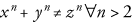# Unit test: informalequation.001

As an image first.Equation 1Fermat's Last Theorem

But this one actually works better as a `mathphrase`. Or at least it works if you have a reasonable set of fonts.

xn + yn ≠ zn ∀ n > 2
Equation 2Fermat's Last Theorem